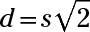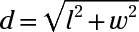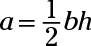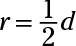##### ASVAB AFQT For DummiesThe math subtests of the ASVAB often ask you to use basic geometry formulas to calculate geometric measurements. You should commit these simple formulas to memory if you are hoping for a high score.

## Quadrilateral formulas you should know

You may be asked to calculate the length of the perimeter, the area, or the diagonal of a square or rectangle. Use the following formulas:

• Perimeter of a square:p = 4s, where s = one side of the square

• Area of a square:a = s2

• Diagonal of a square:• Perimeter of a rectangle:p = 2l + 2w, where l = the length and w = the width of the rectangle

• Area of a rectangle:a = lw

• Diagonal of a rectangle:## Good-to-know triangle formulas

Some math problems on the ASVAB may ask you to calculate the perimeter or area of a triangle. The following formulas are used for these two purposes:

• Perimeter of a triangle: p = s1 + s2 + s3, where s = the length of each leg of the triangle

• Area of a triangle:where b = the length of the triangle’s base (bottom) and h = the height of the triangle

A special formula called the Pythagorean theorem says that if you know the length of any two sides of a right triangle, you can find the length of the third side. It only works on right triangles, however. The formula is a2 + b2 = c2, where c equals the length of the triangle’s hypotenuse and a and b equal the lengths of the remaining two sides.

## Circle formulas

Circles are a bit more complex than squares, rectangles, and triangles and often involve invoking the value of π. Remember that π is approximately equal to 3.14.where d = the diameter of the circle

• Diameter of a circle: d = 2r

• Circumference of a circle: c = 2πr

• Area of a circle: a = πr2

## Handy formulas for three-dimensional shapes

Sometimes the math subtests require you to calculate measurements for solid (three-dimensional) shapes. These types of questions generally come in two flavors: calculating volume or calculating surface area.

Volume is the space a shape takes up. You can think of volume as how much a shape would hold if you poured water into it. Surface area is the area of the outside of the shape — for example, the amount of area you’d have to cover if you were to paint the outside of the solid shape.

• Volume of a cube: v = s3, where s = the length of one side of the cube

• Volume of a rectangular box: v = lwh, where l = the length, w = the width, and h = the height of the box

• Volume of a cylinder: v = πr2h, where r = the radius of the cylinder and h = the height of the cylinder

• Surface area of a cube: SA = 6s2

• Surface area of a rectangular box: SA = 2lw + 2wh + 2lh